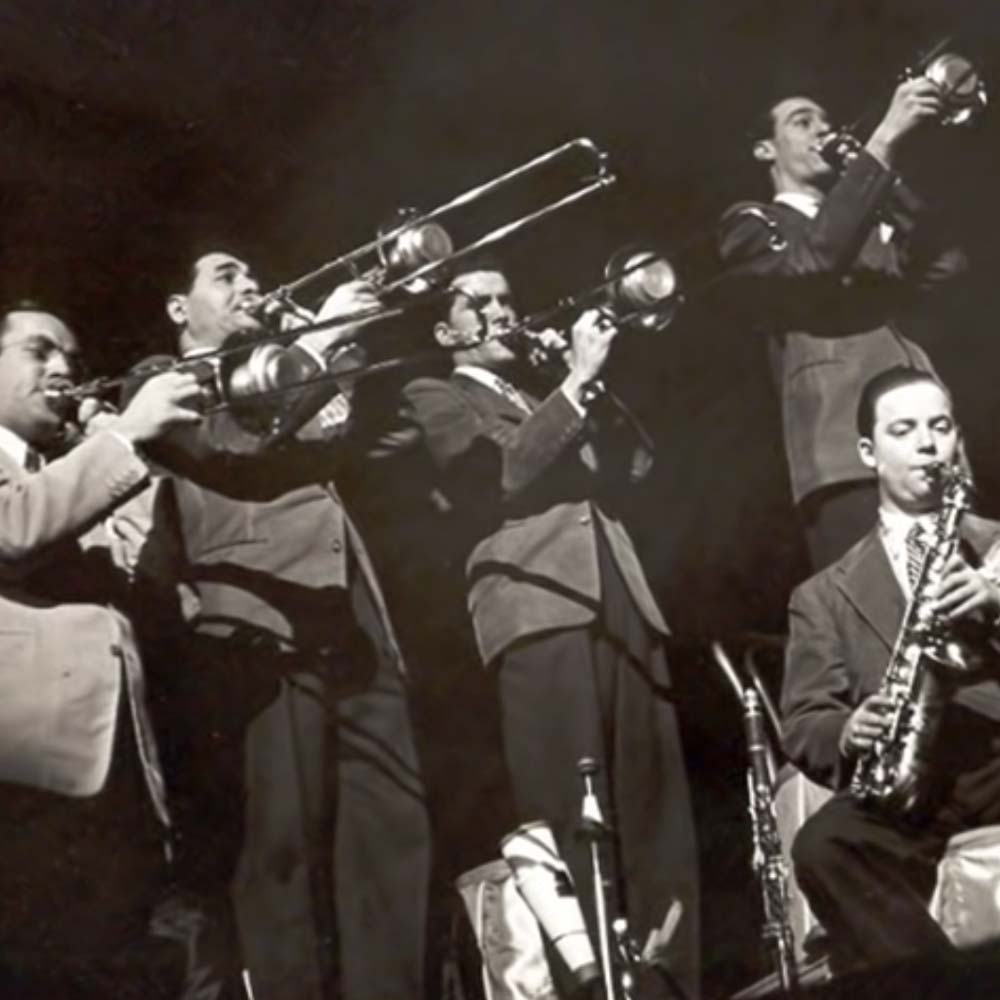# Little Brown Jug

1 July 2021
4
• ##### TARGET LENGTH
00:50
0
(0)

o|
[8u] o o o
[9i] p p p p
[wa] a a p a
[8s] d f
[8u] o o o
[9i] p p p
[wa] a p a
[8s] o s
[8o] o o
[qp] p p
[9a] a a p a
[8s] d f
[8o] o o
[qp] p p
[9a] a a p a
[8s] [wo] [8s]

Rate This Music Sheet:

Average rating 0 / 5. Vote count: 0

No votes so far! Be the first to rate this music sheet.

Thank you for rating this song!

If you have any specific feedback about how to improve this music sheet, please submit this in the box below.

Little Brown Jug is a song by Joseph Eastburn Winner. Use your computer keyboard to play Little Brown Jug music sheet on Virtual Piano. This is an Easy song and requires practice. The recommended time to play this music sheet is 00:50, as verified by Virtual Piano legend, Mark Chaimbers. The song Little Brown Jug is classified in the genre of USA on Virtual Piano. You can also find other similar songs using Folk.

• time
user
Rating
ac.
rm.
score

## Related

•7 7 7 6 7 9 7 \$
6 7 7 7 6 7 q 0
9 6 7 7 7 6 7 9
7 \$ 6 7 7 7 6 7
q 0 9 6 7 7 7 6
7 9 7 \$ 6 7 7 7
6 7 q 0 9 6 7 7
7 6 7 9 7 \$ 6 7
7 7 6 7 r r r e
r y r Q e r r r
e r i u y e r r
r e r y r Q e r
r r e r i u y e
r r r e r y r Q
e r r r e r i u
y e r r r e r y
r Q e r r r e r
7 * 9 0 Q 7 9 e
W Q 0 Q 7 * 9 0
Q 7 9 e W e r W
Q 7 * 9 0 Q 7 9
e W Q 0 Q 7 * 9
0 Q 7 9 e r e W
Q 9 * 7 6 7 7 \$
6 7 * 6 7 9 * 7
6 7 7 \$ * 9 * 6
3 y T r e r r Q
e r T e r y T r
6 9 Q 0 ( 7 e W
Q 9 * 7 6 7 6 7
6 7 6 7 7 * 9 0
Q 9 9 0 q 0 9 *
9 0 Q Q r 7 * 9
0 9 * e w Q 9 9
0 q 0 9 * 9 0 Q
Q r 7 * 9 5 \$ 4
9 ^ 7

Level: 3
Length: 02:46
Easy

#### Yugo Kanno

•l k j h f l k j j h f k j j j h s k j h z l k j h l k j j h f k j j j h s k j h l k j h f l k j j h f k j h j h h l l z z l z z l z z l h z z l z l l x z l x z l k j h l k j j h f k j h j k l
Level: 3
Easy

#### Edith Piaf

•a S a p | S S a p | S |
a S a p | S S a | p S |
a S a p | G G G G f |
da d f | G G G G | f | d | a f |
g g g | f | f d | d |
f f f | d | d s | s |
d d d | s | s a | p | a s |
g g g | f | f d f da |
f f f | d | d s d sp |
d d d | s | s a | p | a s

Level: 1
Length: 02:10
Super Easy